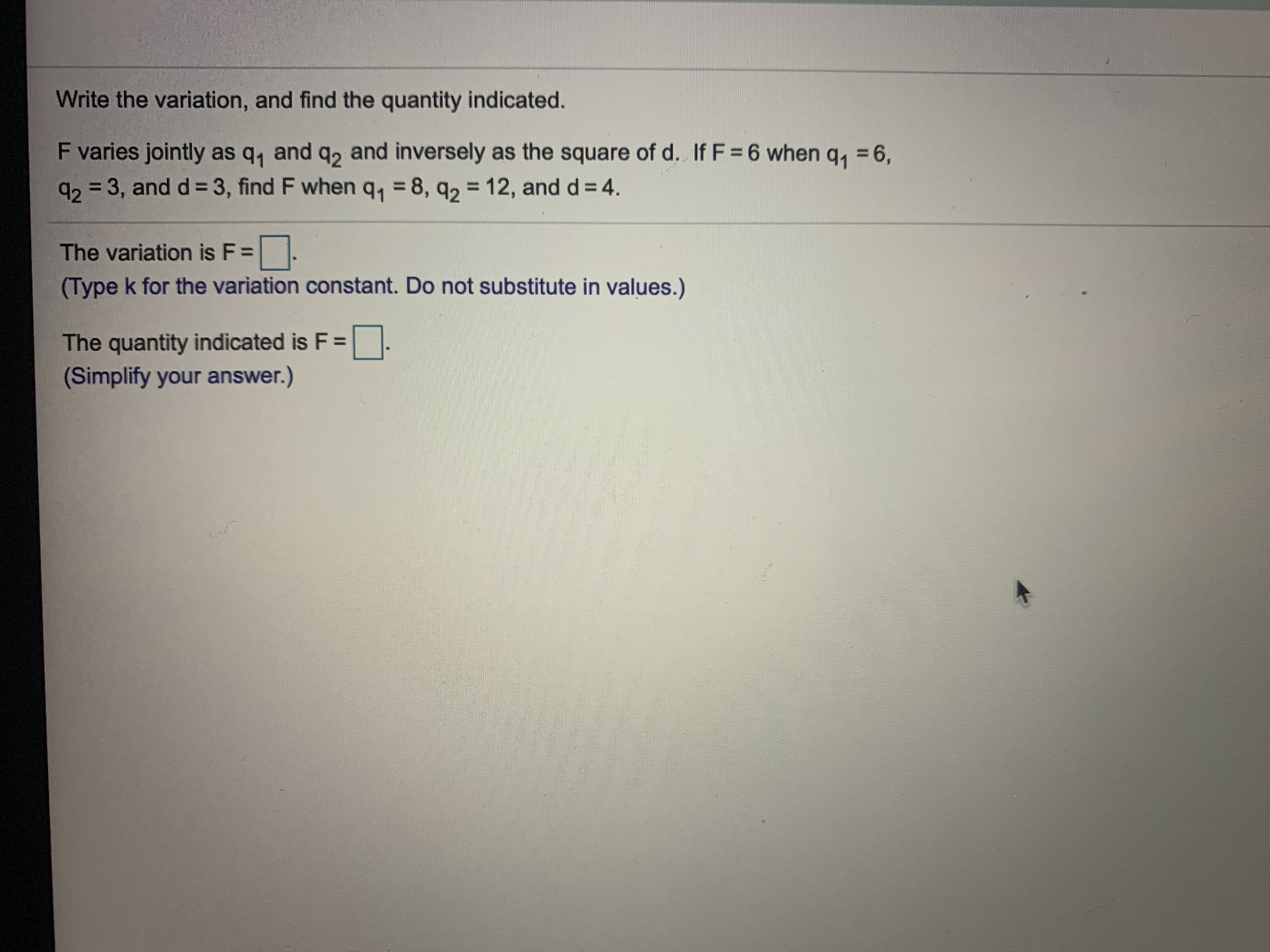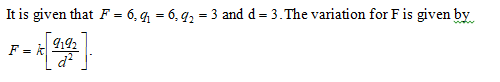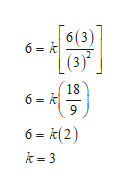Write the variation, and find the quantity indicated.F varies jointly as q, and q2 and inversely as the square of d. If F = 6 when q,q23, and d 3, find F when q1 8, q2 12, and d = 4.6,The variation is F =(Type k for the variation constant. Do not substitute in values.)The quantity indicated is F =(Simplify your answer.)

Questionhelp_outlineImage TranscriptioncloseWrite the variation, and find the quantity indicated. F varies jointly as q, and q2 and inversely as the square of d. If F = 6 when q, q23, and d 3, find F when q1 8, q2 12, and d = 4. 6, The variation is F = (Type k for the variation constant. Do not substitute in values.) The quantity indicated is F = (Simplify your answer.) fullscreen
Step 1Step 2

Evaluate k as,help_outlineImage Transcriptionclose6(3) 6 k (3) 18 6 k 6- (2) k 3 fullscreen
Step 3

Thus, the variation consta...

Want to see the full answer?

See Solution

Want to see this answer and more?

Our solutions are written by experts, many with advanced degrees, and available 24/7

See Solution
Tagged in

Other